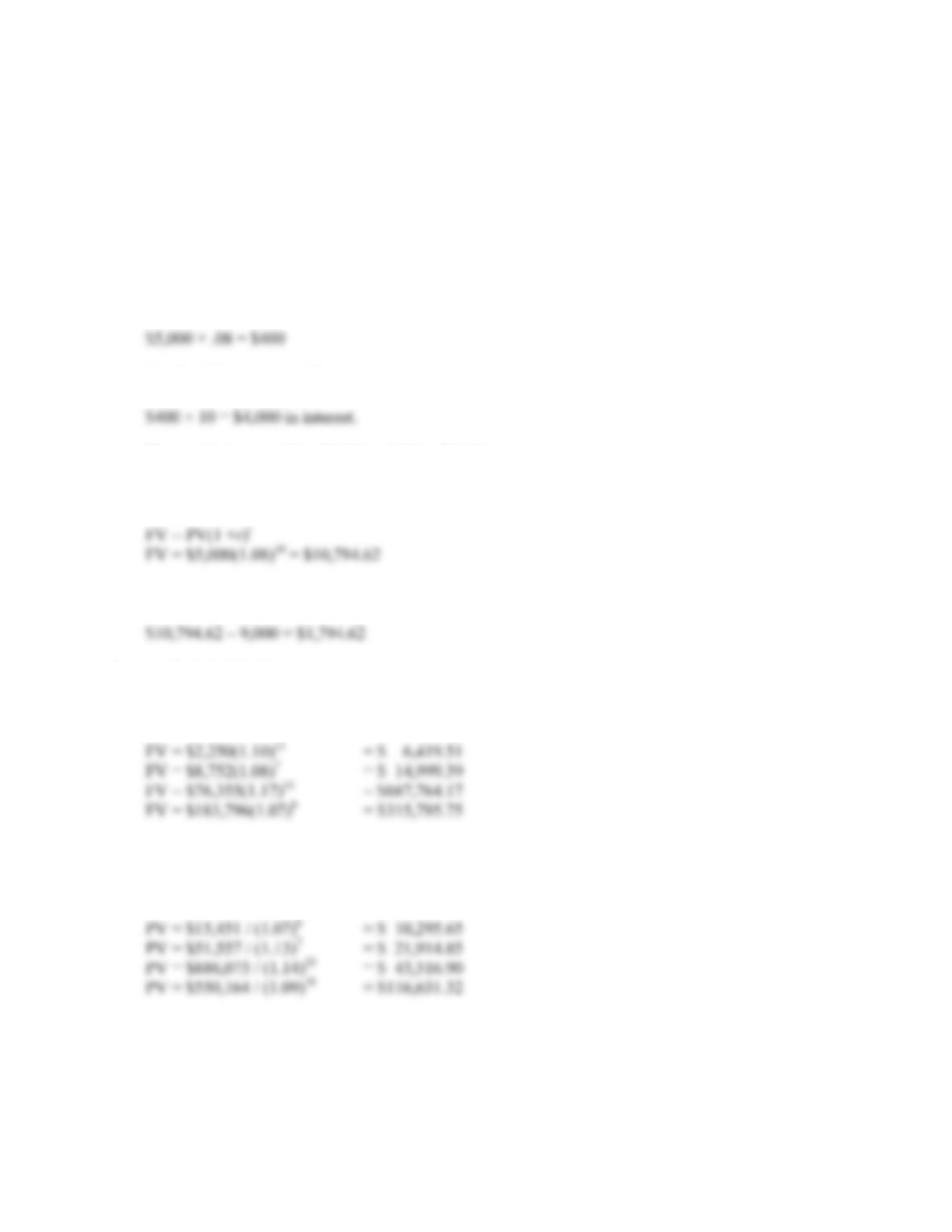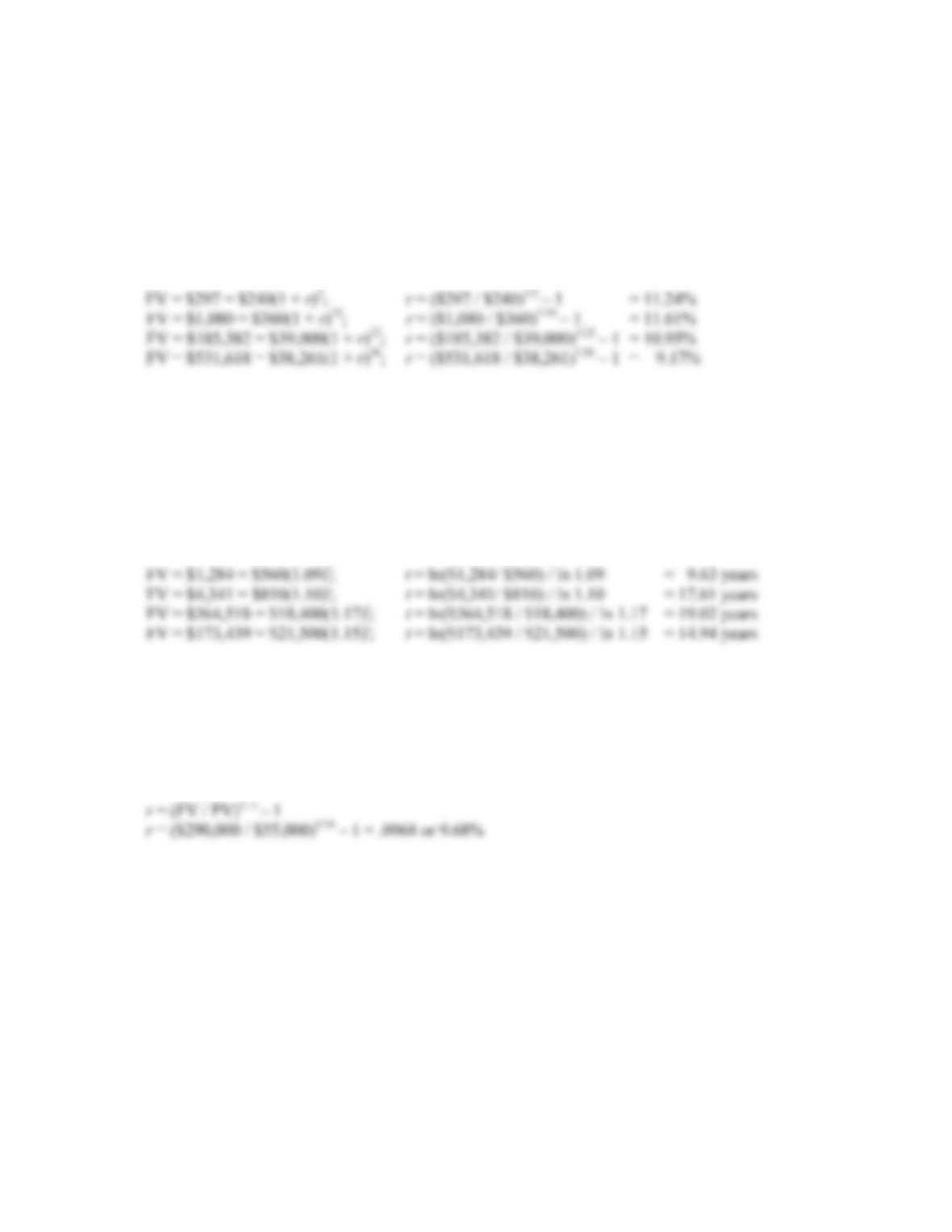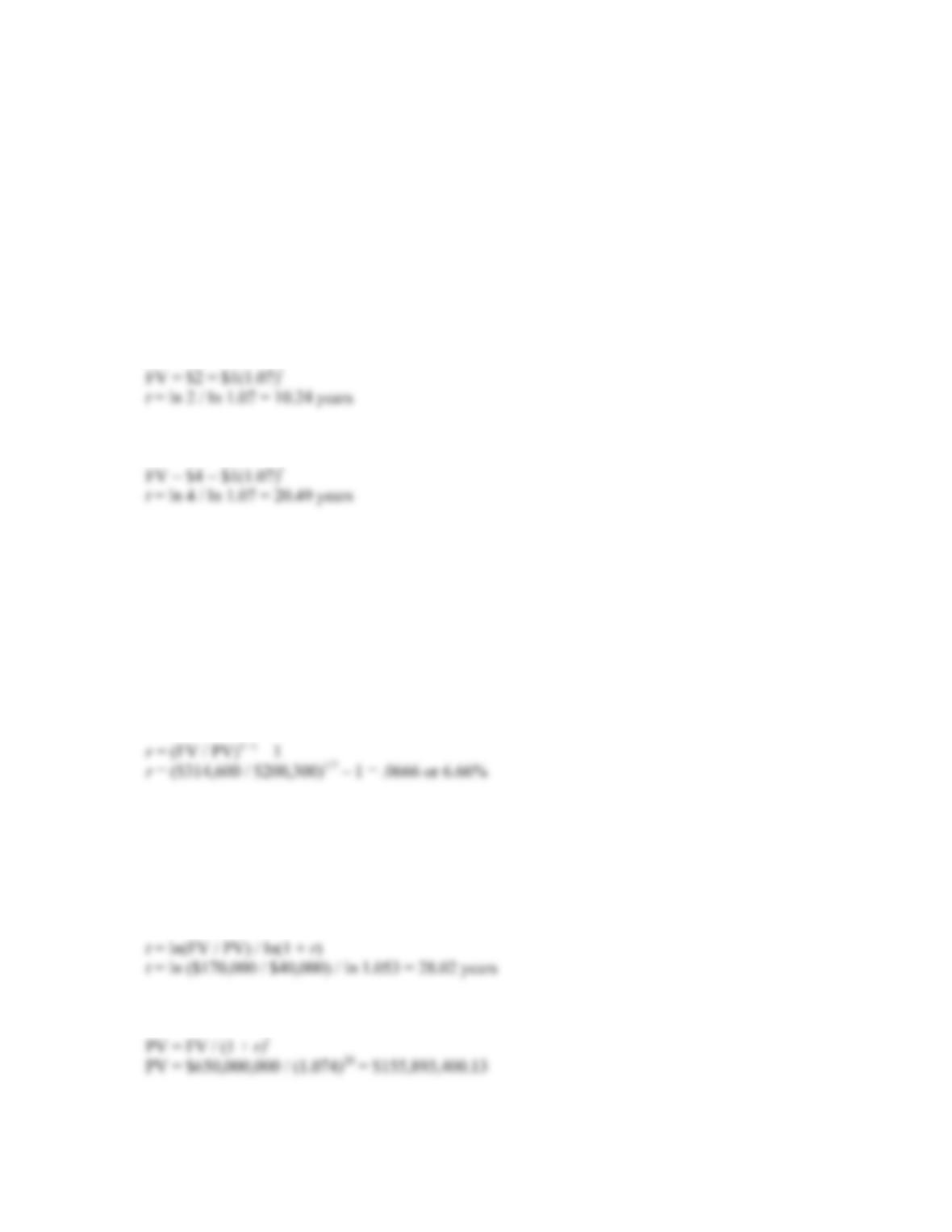Type
Quiz
Book Title
Fundamentals of Corporate Finance Standard Edition 9th Edition
ISBN 13
978-0073382395

### 978-0073382395 Chapter 5 Questions and Problems 1-10

April 3, 2019B-58 SOLUTIONS
Solutions to Questions and Problems
NOTE: All end of chapter problems were solved using a spreadsheet. Many problems require multiple
steps. Due to space and readability constraints, when these intermediate steps are included in this
solutions manual, rounding may appear to have occurred. However, the final answer for each problem is
found without rounding during any step in the problem.
Basic
1. The simple interest per year is:
So after 10 years you will have:
The total balance will be \$5,000 + 4,000 = \$9,000
With compound interest we use the future value formula:
The difference is:
2. To find the FV of a lump sum, we use:
FV = PV(1 + r)t
3. To find the PV of a lump sum, we use:
PV = FV / (1 + r)tCHAPTER 5 B-59
4. To answer this question, we can use either the FV or the PV formula. Both will give the same answer
since they are the inverse of each other. We will use the FV formula, that is:
FV = PV(1 + r)t
Solving for r, we get:
r = (FV / PV)1 / t – 1
5. To answer this question, we can use either the FV or the PV formula. Both will give the same answer
since they are the inverse of each other. We will use the FV formula, that is:
FV = PV(1 + r)t
Solving for t, we get:
t = ln(FV / PV) / ln(1 + r)
6. To answer this question, we can use either the FV or the PV formula. Both will give the same answer
since they are the inverse of each other. We will use the FV formula, that is:
FV = PV(1 + r)t
Solving for r, we get:B-60 SOLUTIONS
7. To find the length of time for money to double, triple, etc., the present value and future value are
irrelevant as long as the future value is twice the present value for doubling, three times as large for
tripling, etc. To answer this question, we can use either the FV or the PV formula. Both will give the
same answer since they are the inverse of each other. We will use the FV formula, that is:
FV = PV(1 + r)t
Solving for t, we get:
t = ln(FV / PV) / ln(1 + r)
The length of time to double your money is: